# Probability for Machine Learning #1 (basics part 1)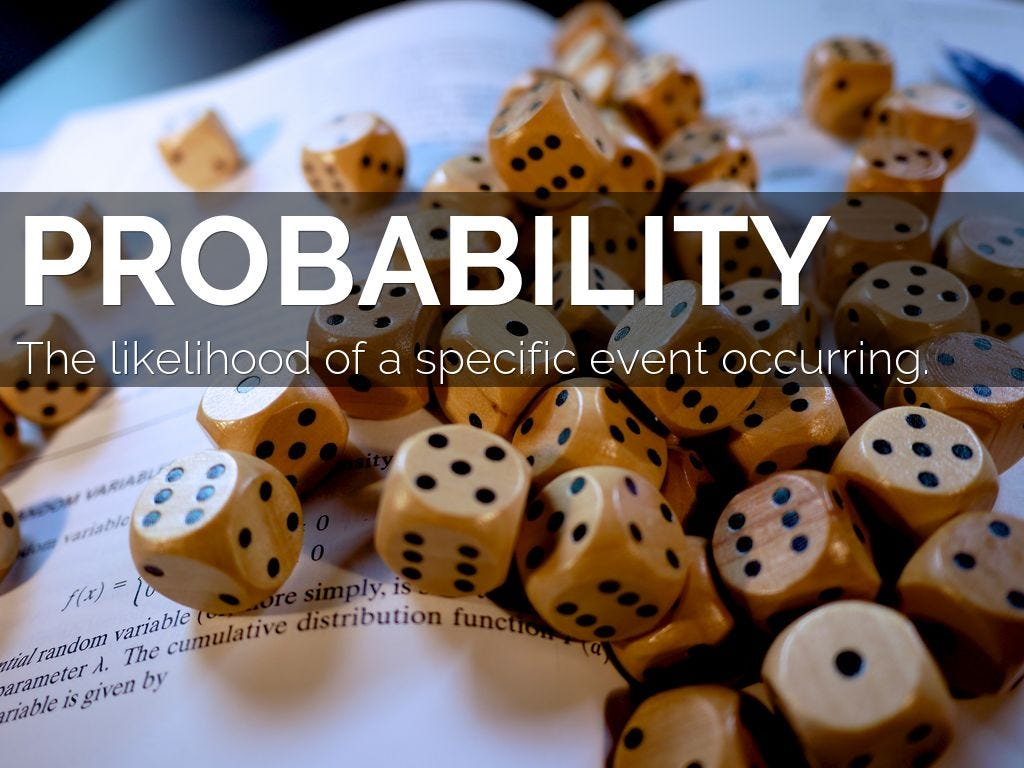Image courtesy (Google)

# Introduction:

I am really glad to study this series trying my best to complete the full mathematics and statistics for machine learning for beginners, starting from the probability to stats, linear algebra, calculus, optimization theory, in a full byte sized form (with well furbished hand written notes ). It may take a lot of time, but I guess readers here would collaborate with me, and also please comment so that I can improve more in the next time.

# Disclaimer

I really assume that readers here know the basics of probability and sets i.e. what is probability, how to find the probability of certain events, also I assume that readers knows the basics of sets, i.e. what are they, union, intersections of sets and other basics of sets, which would be enough to start this series. So lets start without any further due lets start.

# Contents

→ Probability spaces
→ Example to understand sigma algebra, event spaces, probability spaces
→ The fundamental formulas of probability
→ Probability measure
→ Conditional Probability
→ Independence in probability
→ Joint and marginal probability
→ References

# Probability Spaces

Before defining what is probability spaces, the first thing to come in mind is what is a space in a mathematics, because you may have heard or you all will hear about the term called vector spaces , etc. Informally a space is basically defined as a structure where all the similar types of things are kept, which in the case of mathematics can be real or abstract things . For eg, collecting similar types of flower and keeping to a different bags where each bag defines a specific type of flower defines a space of flowers. Similarly abstract things like any event can also be grouped together in a space, like the weather of the day, or whether your friend will come on the right time or not (which are all some example of some abstract intangible events but together grouped in a certain way) , In the terms of probability we define each of the occurrences (like the rain, sunny or windy ) as events , all the things viz {sunny, windy, rainy, cold} are to be said as an event space, and the space where all the possibilities are kept together {sunny, rainy, windy, cold, haze, null } is said to be the sample space (it will contain all the possibilities, that may or may not contain in the event spaces). And each of the events has some probability for its occurrences

## So a probability space is defined as the mathematical space containing three basic components, a sample space (Ω) , an event space (F) , and a probability measure (P)

Please see the picture below to have more clarity on this topic

sample space (Ω) The sample space contains the set of all the possible events including the null event (represented as Φ )

an event space (F) The event space is basically the subset under the sample space (Ω), that must come under the field of sigma algebra, and following some condition as shown below.

Probability Measure (P) It is the fundamental function calculating the probability for the specific event w.r.t to the other all events.

The detailed explanation is shown below.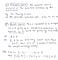Fig 1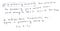Fig 2

# Example to understand sigma algebra, event spaces, probability spaces

Let us assume that the school teacher classified the students of the class with some marks distribution as follows:
if 40 < marks < 50, then the student contains low marks (L)
if 50≤ marks ≤ 80 , then the students will considered as medium (M)
if 81≤ marks ≤ 100 , then the students will be considered as Good (H)

Now for a random students , if the teacher want to predict about the students then he/ she have to first have to make some sample spaces, then have to look after the event he/she is wanting to look for (here , the marks vs the students) and calculate the respective probability.

Now the picture below will make the problem statement more clear, and will show you the event spaces of the total scenario

So all the possible events here are:

if the students contains low or medium or high marks (L U M U H)
if the students contains low or medium (L U M)
if the students contains medium or high marks (M U H)
if the students contains low or high marks (L U H)
if the students contains low (L)
if the students contains medium (M)
if the students contains only high marks (H)
if the students is new, i.e. has no marks in his/her profile (Φ)

Here each of the events are a subset under the sample space.

Let the complement of an event (A) is represented as comp(A)
So here the event space (F) is a σ-algebra, coz for any event , the complement of the event also exist.
for eg. the event the student get marks between 50 to 80 exist, and the same time the event that the student didn’t get marks between the range of 50 to 80, i.e. the student may get marks less than 50 under the category Low(L) or above 80 (under High category). The union of the events also belongs to F. The null event i.e. the student may not have given any exam or a new student is there also belongs(∈) F. So it follows all the basic requirements to fall under σ-algebra. Hence this is how we define a sample space , event space for any problem statement like this.

# The Fundamentals of probability and some formulas

Let A, B be two events such that (A, B ∈ F), also it is given that the events A, B are disjoint to each other, i.e. the events A, B are not at all dependent to each other at any ways and (A∩B = Φ) then we can say as follows: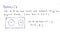Fig 4

So if (A∩B = Φ) then P(A∩B) = P(Φ) = 0
where the P denotes the probability function i.e. the probability of an arbitrary event A is P(A).

Then in order to find the probability of occurrence of event A or B i.e. P(AUB) can be shown as follows: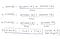Fig 5

Now there can be chance that the two events A, B may not be disjoint i.e.
A∩B Φ, then we can define the probability of occurrence of A or B can be shown as follows: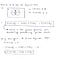Fig 6

So I guess now the concept of how to find the events and their union , intersection can be found very easily.

# Probability measure

If we have to understand it easily the , its simply the general representation to find probability of n-events which ∈ F, such that any random event are disjoint to each other, then we can define this as follows as shown: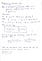Fig 7

The events we are talking here all belonging to F, as a subset of the sample space, and the probability measure for a sample space is always equal to 1.

# Conditional Probability

Mathematically speaking let S1, S2 be two events such that they are mutually dependent to each other, which means basically for event S1 to occur S2 has to occur in some ways or other and vice versa. This concept totally updates the modelling the problems of probability.

So mathematically represented as P(S2|S1) or P(S1|S2)
The representation P(S2|S1) means that probability that the event S2 will occur given that event S1 has already occurred.

It can be seen as follows: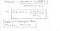Fig 8

# Independence in probability

Two events A, B are said to be independent if and only if , Probability of one event let say A, doesn’t affect the probability that event B to occur, Please don’t get disjoints and independent events. Disjoint events are those events where the intersection is null but for an independent event the intersection is not null.

The independent relationship of two probability is shown as follows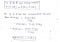Fig 9

# Joint and Marginal Probability

This is todays last topic, so in order to get this concept , I first want to give you a problem statement as follows.

Recently a survey was taken in my college CSE section , of the number of students girls, boys differently about the game they like to play and we get the data as follows: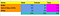Fig 10

Now there are some questions given to us as follows (and don’t think girls don’t play games….😁😁😎)

The questions are as follows, find:

1. P(Players loving to play PUBJ and are females)
2. P(Players playing only PUBJ)
3. P(Players playing are male)
4. P(Players are playing either GTA5 or are male)

So after seeing this table we came to this following inferences and observations as shown below:

Now computing the answers here we will simultaneously get to know get our previous concepts clear.
So lets goooo….

The answer of the first question is shown below: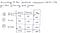Fig 11

Here Po, C, G are representation of the games in short form for simplicity

Now dividing the full data frame by the total number of students we get, the same data frame as follows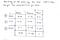Fig 12

Here the each if the cells belonging to the male, female column are the joint probabilities , as each of the cells in those two columns represent the P(playing a certain game by a gender). for eg. the first cell of male column represents the P(players are playing PUBJ and are male) Similarly the other cells of those two columns(except the last column) represents the same.

So the total two columns male, female and their first three columns are together to be said as the joint probability distribution.

Similarly the last column named as “total” and the first three rows are said to be the marginal probability distributions and the each cell of that column is said to be marginal probability.

The below picture would make the concept more clear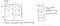Fig 13

Now answering the questions

## answer 1:

As the first question tells to find the P(players playing PUBJ and are female) is specifically the cell of first row of the female column , as the joint probability. So we can write the same thing as follows: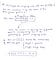Fig 14

## Answer of the second question

P(players playing PUBJ), Here the question didn’t asks about the gender, so specifically we have to find the
P(players playing PUBJ and are male)+P(players playing PUBJ are female),
which we can visualize the same thing given below more elaborately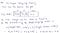Fig 15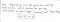Fig 16

## Answer to the third question

P(players playing games are male), So here we do not have to care about female playing games, only males, which becomes equivalent as :
P(players playing are male and playing PUBJ) + P(players playing are male and playing COD) + P(players playing are male and are playing GTA5)
and all the probabilities broken down here are already present in the table.
The pic below makes it more clear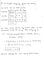Fig 17

## Answer of the final question

P(Players playing GTA5 or are male), The answer of this question can be done in two ways , one is by direct formulas, and other is intuitively. Lets start with direct formula

Mathematically we need to find P(G U M) , in order to find this if we know that P(G ∩ M) then with the formula
P(G U M) = P(G) + P(M) — P(G ∩ M), we can easily compute the answers and the details of this shown below.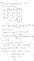Fig 18

Now we can also do it intuitively with the concepts of joint probability and marginal probability distributions

As here it is needed that we need to compute that
P(Players playing GTA5 or are male), then there is no direct relation with females, so if we sum up the first three rows of the male column , which will denote the total probability of male playing games and the sum of the male , female column of the GTA5 row, denoting the probability of players playing GTA5 and minus the common cell of Male column and row GTA5, as we are summing that value(0.1) two times, then we can easily get the value of what we need actually

The same thing told above is shown below: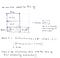Fig 19

The same thing cab also be done with the help of the marginal probability distribution concept, by the same way done before, the pic below would make this more clear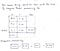Fig 20

So this is all about the topics we discussed today, this are the building blocks for the topics that would be discussed in the next article, containing the concepts of Conditional Independence, Bayes theorem, and full analysis of a real world small problem, which would be fun, and all completes the basic and the foundation for probability. The next article would be coming the next week, so stay tuned.

So Congratulations, 🤩🤩🥳🥳😁😁😀 to complete the first article of the series and now you can
easily:
→ understand the notations and the theories related to the probability spaces
→ know what is probability measure
→ know what is conditional probability
→ know what is joint and marginal probability distributions
→ solved a problem based on the concepts learned today.

Thank you for today, till stay tuned for the next article…😁

# References:

→ YouTube
Probability stats for data science (PDF)

18 years old guy Mathematics , deep learning, machine learning, beats and music , and some fun , is what all about... our life's reinforcement learning...

## More from Anindyadeep

18 years old guy Mathematics , deep learning, machine learning, beats and music , and some fun , is what all about... our life's reinforcement learning...